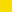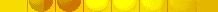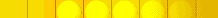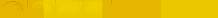An Optimal Solution for the 8x8 Diagonal Solitaire Puzzle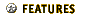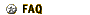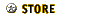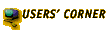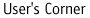• Free Games | Patches | Discussion Board | Discoveries

Note: To find this game in Zillions of Games click the "Square Solitaire" tile and then choose "8x8 Diagonal Solitaire" from the Variant menu.

### BACKGROUND

In "8x8 Diagonal Solitaire" 48 marbles are initially arranged on an 8x8 grid as folows:

```OOOOOOOO
OOOOOOOO
OO::::OO
OO::::OO
OO::::OO
OO::::OO
OOOOOOOO
OOOOOOOO

diagram 1
```

Marbles jump diagonally as in Checkers and the jumped-over marble is removed. The goal is to reduce the position to as few marbles as possible.

In "BASIC Computer Games" (1978) David H. Ahl writes that "It is easy to remove 30 to 39 checkers, a challenge to remove 40 to 44, and a substantial feat to remove 45 to 47." Anthony S. Fipiak writes in "Mathematical Puzzles and Other Brain Twisters" (1942) that "To have one peg on board is a very difficult feat." Neither author furnishes a solution. It turns out that the substantial/difficult feat they talk about is an impossibility. The best solution possible is a removal of 44 marbles, leaving 4 marbles remaining on the board.

### THE PROOF

If the board were checkered, then marbles would never leave their own color since only diagonal jumps are allowed. Thus the problem is really two instances of the problem of reducing all the marbles on a single color:

```O:O:O:O:
:O:O:O:O
O:::::O:
:O:::::O
O:::::O:
:O:::::O
O:O:O:O:
:O:O:O:O

diagram 2
```

If you look at diagram 2 diagonally, tilting it clockwise, you see that this problem is equivalent to the standard type (orthogonal jump) solitaire puzzle shown in diagram 3. All the positions of the "other color" have been removed.

```   O
OOO
OO:OO
OO:::OO
OO:::OO
OO:OO
OOO
O

diagram 3
```

Reducing it to a standard type of puzzle means we can use John D. Beasley's analysis ("The Ins and Outs of Peg Solitaire", chapter 4) on it. First we label the diagonals as in diagrams 4 and 5:

```   A
ABC
ABCAB
ABCABCA
BCABCAB
ABCAB
CAB
B

diagram 4

D
EFD
FDEFD
DEFDEFD
FDEFDEF
FDEFD
FDE
F

diagram 5
```

and count the squares that have men on them initially:

```Diagonal A 10 (even)
Diagonal B 10 (even)
Diagonal C  4 (even)
Diagonal D 10 (even)
Diagonal E  4 (even)
Diagonal F 10 (even)
--------------------
Total men: 24 (even) ```

In Beasley's terminology the position is "in phase" for every diagonal, since each diagonal has the same parity (even) in the number of men as the total. As moves are played the parities change, but the phase relationships do not. Therefore in order for there to be a single marble solution, diagonals A-F would all have to have odd parity. Since this is not possible, there is no single marble solution.

In practice it offen occurs that an endgame is reached with three marbles in a row. For example, a horizontal line just above the center would have the marbles on CAB and FDE. This is a plausible position since the total (3) has odd parity as does every diagonal (1). Now if a jump is made to the right you are left with two marbles. The total (2) has an even parity as do the resulting diagonals: A,B,D,E (0) and C,F (2). Since a single marble solution doesn't exist, reducing down to two marbles like this is the best you can do.

If a two marble solution is the best that can be done for a single square color, the minimum solution that can be achieved for the original puzzle is four marbles (two on each color).

### SAMPLE 4-MARBLE SOLUTION

This is a sample 4-marble solution. First one color is solved and then the other color is solved symmetrically. The output is taken from a "Zillions of Games" saved game file. Algebraic chess coordinates are used.

```1. Blue a8 - c6 x b7
2. Blue a6 - c4 x b5
3. Blue c8 - e6 x d7
4. Blue b1 - d3 x c2
5. Blue d3 - b5 x c4
6. Blue a2 - c4 x b3
7. Blue f7 - d5 x e6
8. Blue c6 - e4 x d5
9. Blue a4 - c6 x b5
10. Blue f1 - d3 x e2
11. Blue h1 - f3 x g2
12. Blue f3 - d5 x e4
13. Blue c6 - e4 x d5
14. Blue c4 - e2 x d3
15. Blue d1 - f3 x e2
16. Blue h5 - f7 x g6
17. Blue g8 - e6 x f7
18. Blue f3 - d5 x e4
19. Blue d5 - f7 x e6
20. Blue e8 - g6 x f7
21. Blue h7 - f5 x g6
22. Blue g4 - e6 x f5
23. Blue a1 - c3 x b2
24. Blue a3 - c5 x b4
25. Blue c1 - e3 x d2
26. Blue b8 - d6 x c7
27. Blue d6 - b4 x c5
28. Blue a7 - c5 x b6
29. Blue f2 - d4 x e3
30. Blue c3 - e5 x d4
31. Blue a5 - c3 x b4
32. Blue f8 - d6 x e7
33. Blue h8 - f6 x g7
34. Blue f6 - d4 x e5
35. Blue c3 - e5 x d4
36. Blue c5 - e7 x d6
37. Blue d8 - f6 x e7
38. Blue h4 - f2 x g3
39. Blue g1 - e3 x f2
40. Blue f6 - d4 x e5
41. Blue d4 - f2 x e3
42. Blue e1 - g3 x f2
43. Blue h2 - f4 x g3
44. Blue g5 - e3 x f4 ```

Next --> Blocking A Wormhole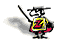About Zillions Development Dealer Inquiries are welcome here. Copyright 1998-2019 Zillions Development Corporation Zillions Privacy Statement